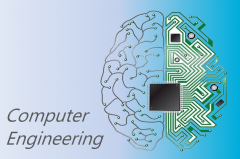• Follow Us# CE-200 - Digital Electronics, Fundamentals## CE-200 - Digital Electronics, Fundamentals

This course introduces the students to the basics of digital logic circuits. The course starts with a description of binary representation of numbers, binary math and digital representation of binary numbers. Digital logic gates are introduced next leading to and introduction to combinatorial logic simplification techniques and Karnaugh maps. In any modern logic circuit design, power consumption is of vital importance. The course, therefore, leads the students through an understanding of logic circuit power consumption analysis and battery life estimation.